## Name

ST_Overlaps — Tests if two geometries have the same dimension and intersect, but each has at least one point not in the other

## Synopsis

`boolean ST_Overlaps(`geometry A, geometry B`)`;

## Description

Returns TRUE if geometry A and B "spatially overlap". Two geometries overlap if they have the same dimension, their interiors intersect in that dimension. and each has at least one point inside the other (or equivalently, neither one covers the other). The overlaps relation is symmetric and irreflexive.

In mathematical terms: ST_Overlaps(A, B) ⇔ ( dim(A) = dim(B) = dim( Int(A) ⋂ Int(B) )) ∧ (A ⋂ B ≠ A) ∧ (A ⋂ B ≠ B)This function automatically includes a bounding box comparison that makes use of any spatial indexes that are available on the geometries. To avoid index use, use the function `_ST_Overlaps`.

Performed by the GEOS module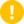Enhanced: 3.0.0 enabled support for `GEOMETRYCOLLECTION`

NOTE: this is the "allowable" version that returns a boolean, not an integer.This method implements the OGC Simple Features Implementation Specification for SQL 1.1.

s2.1.1.2 // s2.1.13.3This method implements the SQL/MM specification.

SQL-MM 3: 5.1.32

## Examples

`ST_Overlaps` returns `TRUE` in the following situations: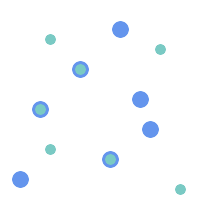`MULTIPOINT` / `MULTIPOINT`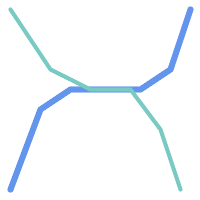`LINESTRING` / `LINESTRING`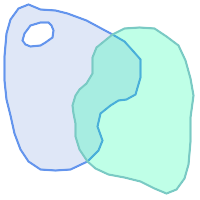`POLYGON` / `POLYGON`A Point on a LineString is contained, but since it has lower dimension it does not overlap or cross.

```SELECT ST_Overlaps(a,b) AS overlaps,       ST_Crosses(a,b) AS crosses,
ST_Intersects(a, b) AS intersects,  ST_Contains(b,a) AS b_contains_a
FROM (SELECT ST_GeomFromText('POINT (100 100)') As a,
ST_GeomFromText('LINESTRING (30 50, 40 160, 160 40, 180 160)')  AS b) AS t

overlaps | crosses | intersects | b_contains_a
---------+----------------------+--------------
f        | f       | t          | t
```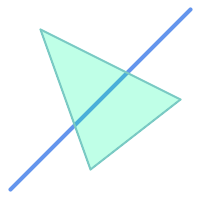A LineString that partly covers a Polygon intersects and crosses, but does not overlap since it has different dimension.

```SELECT ST_Overlaps(a,b) AS overlaps,        ST_Crosses(a,b) AS crosses,
ST_Intersects(a, b) AS intersects,   ST_Contains(a,b) AS contains
FROM (SELECT ST_GeomFromText('POLYGON ((40 170, 90 30, 180 100, 40 170))') AS a,
ST_GeomFromText('LINESTRING(10 10, 190 190)') AS b) AS t;

overlap | crosses | intersects | contains
---------+---------+------------+--------------
f       | t       | t          | f
```Two Polygons that intersect but with neither contained by the other overlap, but do not cross because their intersection has the same dimension.

```SELECT ST_Overlaps(a,b) AS overlaps,       ST_Crosses(a,b) AS crosses,
ST_Intersects(a, b) AS intersects,  ST_Contains(b, a) AS b_contains_a,
ST_Dimension(a) AS dim_a, ST_Dimension(b) AS dim_b,
ST_Dimension(ST_Intersection(a,b)) AS dim_int
FROM (SELECT ST_GeomFromText('POLYGON ((40 170, 90 30, 180 100, 40 170))') AS a,
ST_GeomFromText('POLYGON ((110 180, 20 60, 130 90, 110 180))') AS b) As t;

overlaps | crosses | intersects | b_contains_a | dim_a | dim_b | dim_int
----------+---------+------------+--------------+-------+-------+-----------
t        | f       | t          | f            |     2 |     2 |       2
```